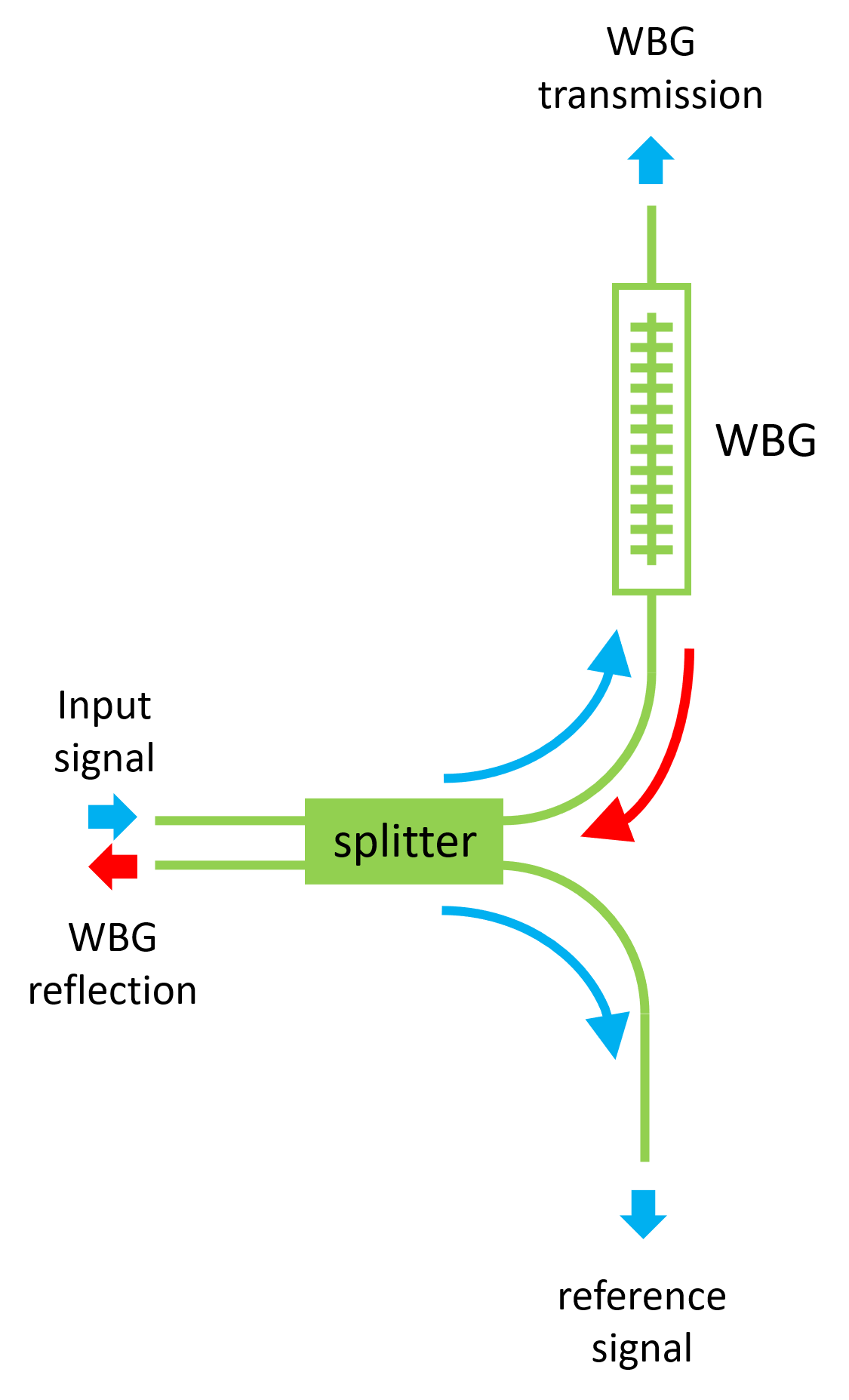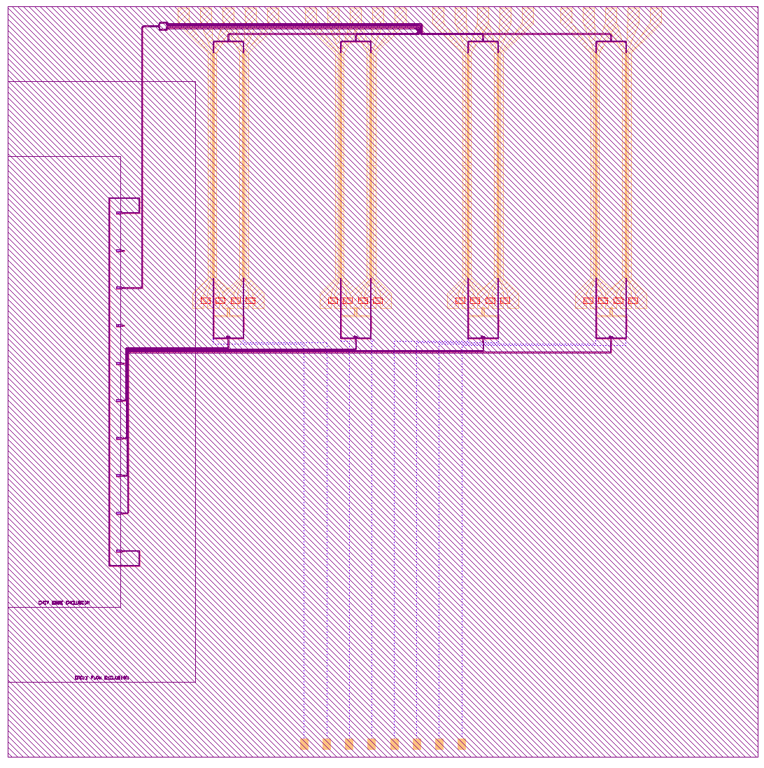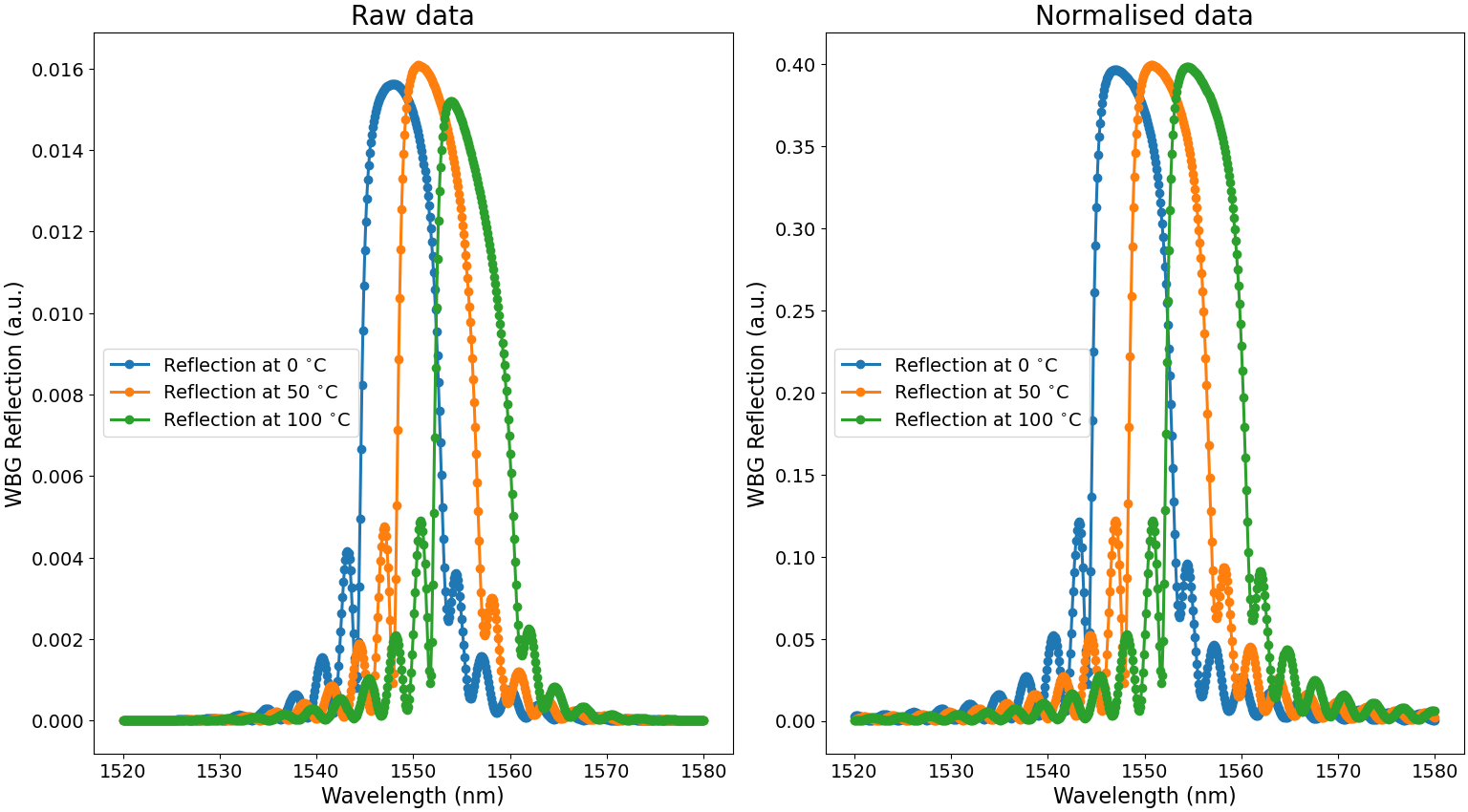# 2.5. Adding fiber couplers: the final working circuit¶

In the previous sections, we have learned how to optimize the waveguide Bragg grating (WBG) to achieve maximal temperature sensitivity. Of course, the WBG cannot function on its own; it needs to communicate with an off-chip laser and detector. For this reason, we still need to connect input and output fiber grating couplers to the WBG. Secondly, we are interested in measuring the reflected signal from the WBG. Since this reflected signal will end up at the input grating coupler, we will need to use an external optical circulator in order to separate the forward propagating signal (the input light) from the reflected signal. Alternatively, we can also route part of the reflected signal on chip to another fiber grating coupler, thus obviating the need for an optical circulator to capture this signal. This is the strategy we decide to follow. Finally, since the input and output fiber grating couplers introduce a certain insertion loss and bandwidth limitation, we need to find a way to normalize the measured WBG spectra with the characteristic of these I/O ports.

Creating a functional circuit is thus the focus of this section, and we will dive into three topics:

1. Creating the circuit layout
2. Simulating the full circuit
3. Export the circuit to a gds file

## 2.5.1. Creating the circuit layout¶

As explained in the introduction, laser light or another light source is required to characterize the WBG. To couple this light into the WBG, we need fiber grating couplers as I/O ports. The SiEPIC PDK provides a pre-designed and tested fiber grating coupler that we can use for this purpose. This building block consists of a specially designed grating that efficiently converts incident light from a fiber into a waveguide mode in a certain wavelength range. Since the fiber is large (diameter of $$125\,\mathrm{\mu m}$$) as compared to the grating coupler (ca. $$10-20\,\mathrm{\mu m}$$ in diameter) and to ease any packaging requirements (if necessary), we will place the grating couplers sufficiently far from each other. The minimum distance that the SiEPIC foundry requires is $$127\,\mathrm{\mu m}$$. Sticking to this distance will also enable the use of fiber arrays, thus greatly simplifying the packaging and experimental characterisation.

The other requirements we mentioned were

• the need to normalise the measured spectra from the WBG due to the limited bandwidth of the I/O grating couplers and
• the need to reroute part of the reflected optical signal to an I/O grating coupler other than the input port.

These two requirements can be met when employing a 2x2-splitter and the functionality is illustrated in Fig. 2.31.Fig. 2.31 Functionality of the temperature sensor circuit.

Light from the input grating coupler will enter one of the input ports of the 2x2 splitter and split in two arms: an arm with the WBG and a reference arm without a WBG. The reflected signal arriving at the output ports of the 2x2 splitter will then be split over the splitter’s input ports; one of the input ports is already connected to the input grating coupler, but the other can be used to reroute part of the reflected signal to another grating coupler. As a result, we have four ports:

1. The input port to couple the light in
2. The output port to measure the transmission spectra of the WBG
3. The reference arm that measures the transmission spectra of two cascaded grating couplers
4. The output port to capture the reflection of the WBG

The reflection spectra from the WBG can thus be normalized to the transmission spectra from the reference arm. The SiEPIC PDK contains several 2x2-splitters. Among those, we choose to use the “adiabatic coupler” 2x2-splitter as it yields a relatively broadband response and has negligible reflections.

We now create a Python file (full_circuit_cells.py) that contains the class FullCircuit, in which we will initiate all the required building blocks. The code is given below:

Listing 2.45 full_circuit_cells.py
class FullCircuit(CircuitCell):

wbg = i3.PCellProperty(doc="Unit cell of the WBG")
fiber_coupler_pitch = i3.PositiveNumberProperty(doc="Distance between fiber grating couplers", default=127.)

def _default_child_cells(self):
fc = pdk.EbeamGCTE1550()
return {
'wbg': self.wbg,
'fib_in': fc,
'fib_out_wbg_t': fc,
'fib_out_ref': fc,
'fib_out_wbg_r': fc,
'floorplan': pdk.FloorPlan(size=(300., 410.))
}

def _default_place_specs(self):
fcp = self.fiber_coupler_pitch
wbg_cell = self.child_cells['wbg']
wbg_length = wbg_cell.Layout().ports['out'].x - wbg_cell.Layout().ports['in'].x
wbg_y_offset = (1.5 * fcp - wbg_length) / 2
fc_names = ['fib_out_ref', 'fib_out_wbg_r', 'fib_in', 'fib_out_wbg_t']
specs = [i3.Place("{}:opt1".format(fc), (0, cnt * fcp)) for cnt, fc in enumerate(fc_names)]
specs.append(i3.PlaceRelative("splitter", "fib_in:opt1", (2 * br, -fcp / 2)))  # noqa
specs.append(i3.PlaceRelative("wbg:in", "splitter:opt3", (br, wbg_y_offset), angle=-90))  # noqa
specs.append(i3.PlaceRelative("floorplan", "fib_out_ref:opt1", (-50, -15)))  # noqa
return specs

def _default_connectors(self):
return [
("fib_in:opt1", "splitter:opt1", manhattan, {'bend_radius': br, 'min_straight': 0}),
("splitter:opt3", "wbg:in", manhattan, {'bend_radius': br, 'min_straight': 0}),
("wbg:out", "fib_out_wbg_t:opt1", manhattan, {'bend_radius': br, 'min_straight': 0}),
("splitter:opt4", "fib_out_ref:opt1", manhattan, {'bend_radius': br, 'min_straight': 0}),
("splitter:opt2", "fib_out_wbg_r:opt1", manhattan, {'bend_radius': br, 'min_straight': 0}),
]

def _default_external_port_names(self):
return {
'fib_in:fib1': 'in',
'fib_out_wbg_t:fib1': 'wbg_t',
'fib_out_wbg_r:fib1': 'wbg_r',
'fib_out_ref:fib1': 'ref',
}


Just like we did with the unit cells in the WBG, we create the instances containing all the necessary building blocks, which for our circuit are:

• Four I/O grating couplers
• One splitter
• One WBG
• Connecting waveguides
• Chip floorplan

This circuit is generated through CircuitCell in a similar way as the splitter tree in Design a circuit: splitter tree, but in which we now use Manhattan bends for the connecting waveguides. We also add the chip floorplan to the circuit, which determines the chip boundaries. Although it is not connected to any functional circuitry (this will give warning when running example_full_circuit.py that can be ignored), it is necessary for the foundry to know where to dice the chip. The input and output ports of the circuit are simply the vertical ports of the I/O grating couplers (fib1), but we rename them accordingly to their function: in, wbg_t, wbg_r and ref.

Now we can simply instantiate and draw the full circuit with the following code in example_full_circuit.py:

Listing 2.46 example_full_circuit.py
width = 0.5
deltawidth = 0.089
dc = 0.2
lambdab = 0.325

uc = UnitCellRectangular(
width=width,
deltawidth=deltawidth,
length1=(1 - dc)*lambdab,
length2=dc*lambdab
)

wbg = UniformWBG(uc=uc, n_uc=300)

full_circuit = FullCircuit(wbg=wbg)
full_circuit_lo = full_circuit.Layout()
full_circuit_lo.visualize(annotate=True)


In this piece of code, we first define the optimized unit cell and we use it to instantiate the WBG PCell. Finally, we pass on this WBG PCell to instantiate the FullCircuit class. The layout is shown here in Fig. 2.32:Fig. 2.32 Full circuit, showing the grating couplers, splitter, WBG arm, reference arm and chip floorplan.

## 2.5.2. Simulating the final circuit¶

The circuit model of our temperature sensor is automatically generated from the layout through CircuitCell. However, it should be mentioned that it is generated for the default temperature, which is 293 K. In order to simulate the circuit at other temperatures, we need to explicitly pass on these temperatures to the circuit model. An example is given at the end of example_full_circuit.py:

Listing 2.47 example_full_circuit.py
temperatures = np.linspace(273, 373, 3)
wavelengths = np.arange(1.52, 1.58, 0.0001)

fig = plt.figure(constrained_layout=True)
gs = gridspec.GridSpec(1, 2, figure=fig)

axes_sparam.set_xlabel(r'Wavelength (nm)', fontsize=16)
axes_sparam.set_ylabel('WBG Reflection (a.u.)', fontsize=16)
axes_sparam.set_title('Raw data', fontsize=20)

axes_sparam.set_xlabel(r'Wavelength (nm)', fontsize=16)
axes_sparam.set_ylabel('WBG Reflection (a.u.)', fontsize=16)
axes_sparam.set_title('Normalised data', fontsize=20)

for temperature in temperatures:

wbg.CircuitModel(temperature=temperature)
full_circuit = FullCircuit(wbg=wbg)

full_circuit_cm = full_circuit.CircuitModel()
full_circuit_Smatrix = full_circuit_cm.get_smatrix(wavelengths=wavelengths)
reflection = np.abs(full_circuit_Smatrix["in:0", "wbg_r:0"])**2
transmission_ref = np.abs(full_circuit_Smatrix["in:0", "ref:0"])**2

axes_sparam.plot(
wavelengths*1000,
reflection,
'o-',
linewidth=2.2,
label=r'Reflection at '+str(int(temperature-273))+r' $^{\circ}C$'
)
axes_sparam.plot(
wavelengths*1000,
reflection/transmission_ref,
'o-',
linewidth=2.2,
label=r'Reflection at '+str(int(temperature-273))+r' $^{\circ}C$'
)

axes_sparam.legend(fontsize=14, loc=6)
axes_sparam.legend(fontsize=14, loc=6)
axes_sparam.tick_params(which='both', labelsize=14)
axes_sparam.tick_params(which='both', labelsize=14)
plt.show()


This script will calculate and plot the reflection spectra, both the raw data from the wbg_r port and the data normalized to the transmission of the reference arm, measured at the ref port. This is done at three different temperatures ($$0^\circ$$, $$50^\circ$$ and $$1000^\circ$$). The results are depicted in Fig. 2.33.Fig. 2.33 Reflection spectra from the WBG. Left: raw transmission data from the wbg_r port. Right: data from the wbg_r port normalised with the transmission measured from the reference arm at the ref port.

Fig. 2.33 illustrates the importance of adding the reference arm. As can be seen, due to the limited bandwidth of the grating couplers, the reflection spectra will get distorted for large temperature changes. This effect can be compensated with normalising the reflection spectra with the measurements from the reference arm as seen in the right plots.

It should be noted that although in reality the behavior of the I/O ports will also change with temperature, it is not taken into account in the grating coupler model as the data was not available at the time of publishing this tutorial. Nevertheless, because we use normalized reflection spectra, the influence of temperature effects in the grating couplers is minimized, once more illustrating the importance of using normalized data in order to obtain reliable measurements.

## 2.5.3. Exporting the final layout to a gds file¶

Now that the full circuit has been designed and numerically characterised, it is finally time to generate the gds file to be sent to the foundry for fabrication. This is very easy to do; simply write

full_circuit_lo.write_gdsii('full_circuit.gds')


at the end of example_full_circuit.py, and the gds-file of the circuit is generated in the directory where example_full_circuit.py is located. You can always check this gds-file in KLayout as depicted in Fig. 2.34.

Now you finally have the layout of a working temperature sensor, ready to be fabricated and packaged by the foundry!